Latest Banking jobs   »   Reasoning Ability Quiz For IBPS Clerk/NIACL...

# Reasoning Ability Quiz For IBPS Clerk/NIACL AO Prelims 2021- 26th August

Directions (1-3): Study the information carefully and answer the questions given below.
Seven persons A, B, C, D, E, F and G are sitting in a circular table Some of them facing towards center and some of them facing away from center but not necessary in same order.
D sits third to the right of B. E sits immediate left of D. Two persons sit between B and G. A is immediate neighbor of C. A sits third to the right of F. Immediate neighbor of G face opposite direction to each other. G face towards the center. Neither G nor C face same direction as D. Not more than four person face towards center.

Q1. Who among the following person sits second to the right of C?
(a) F
(b) D
(c) E
(d) G
(e) None of these

Q2. How many persons sit between A and E, when counted to the right of E?
(a) Two
(b) Three
(c) One
(d) Four
(e) None of these

Q3. How many persons face outside the center?
(a) Four
(b) Two
(c) Three
(d) Five
(e) None of these

Q4. If all the digits in the number “8154276367” arranged in ascending order from left to right then how many numbers remains same in their position?
(a) One
(b) Two
(c) Three
(d) Four
(e) None of these

Q5. If ‘A × D’ mean ‘D is the sister of A’, ‘A + D’ means ‘D is the daughter of A’ , ‘A ÷ D’ means ‘A is the wife of D’, and ‘A-D’ means ‘A is brother of D’ then how will ‘K is mother of P’ be denoted?
(a) J – K ÷ P + L
(b) K + P + M × J
(c) J – K ÷ L + P
(d) K – J ÷ L + P
(e) None of these

Direction (6-10): In these questions, relationship between different elements is show in the statements. The statements are followed by conclusions. Study the conclusions based on the given statements and select the appropriate answer:
(a) If only conclusion I follows.
(b) If only conclusion II follows.
(c) If either conclusion I or II follows
(d) If neither conclusion I nor II follows.
(e) If both conclusions I and II follow.

Q6. Statements: W=E>F>V, M≥W, G≥S≥V
Conclusion: I: E≤M II: M>S

Q7. Statements: H≤R, T<U≤H, H<I<R
Conclusion: I: I≥T II: I>T

Q8. Statements: R>S, P>M≥O, R=Q<O
Conclusion: I: M> R II: S<O

Q9. Statements: B<E<R<T, U≥Y=T, U=X
Conclusion: I: B>Y II: X>E

Q10. Statements: S<M<U, Y>X>R, T≥R, Y<U
Conclusion: I: X>M II: M≥X

Directions (11-15): The following questions are based on the five three – digit numbers given below:

924 574 456 187 675

Q11. If 3 is added to the second digit of each of the numbers how many numbers thus formed will be divisible by three?
(a) None
(b) One
(c) Two
(d) Three
(e) None of these

Q12. If all the digits in each of the numbers are arranged in descending order within the number, which of the following will be the highest number in the new arrangement of numbers?
(a) 924
(b) 574
(c) 456
(d) 187
(e) 675

Q13. What will be the resultant number if the third digit of the second highest number is divided by the 2nd digit of the second lowest number?
(a) 2
(b) 3
(c) 0
(d) 1
(e) 4

Q14. If 3 is added to the first digit and 1 is added to the last digit of each of the number then which of the following numbers will be the second highest number?
(a) 924
(b) 574
(c) 456
(d) 187
(e) 675

Q15. If in each number the first and the third digits are interchanged then which will be the highest number?
(a) 924
(b) 574
(c) 456
(d) 187
(e) 675

Solutions:

Solutions (1-3):
Sol.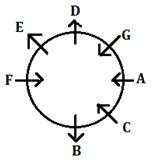S1.Ans(d)
S2.Ans(a)
S3.Ans(c)

S4. Ans(b)
Sol. Original number: 8154276367
Obtained number: 1234566778

S5.Ans(c)
Sol.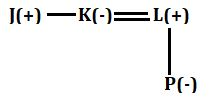S6. Ans(a)
Sol. I: E≤M(true) II: M>S(False)

S7. Ans(b)
Sol. I: I≥T (False) II: I>T (True)

S8. Ans(e)
Sol. I: M>R (True) II: S<O (True)

S9. Ans(b)
Sol. I: B>Y (False) II: X>E (True)

S10. Ans(c)
Sol. I: X>M (False) II: M≥X (False)

S11. Ans. (d)

S12. Ans. (a)

S13. Ans. (d)

S14. Ans. (e)

S15. Ans. (d)#### Congratulations!Incorrect details? Fill the form again here

•Reasoning Ability Quiz For IBPS RRB PO C...
•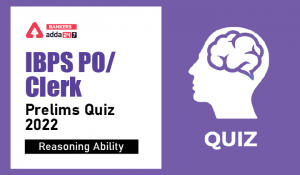Reasoning Ability Quiz For IBPS Clerk/PO...
•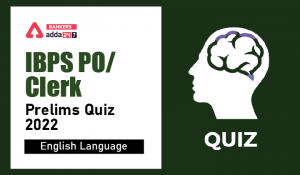English Quizzes For IBPS Clerk/PO Prelim...
•English Quizzes For IBPS Clerk/PO Prelim...
•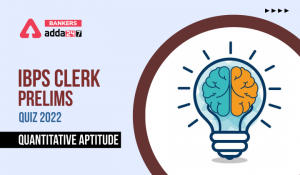Quantitative Aptitude Quiz For IBPS Cler...
•Reasoning Ability Quiz For IBPS RRB PO C...